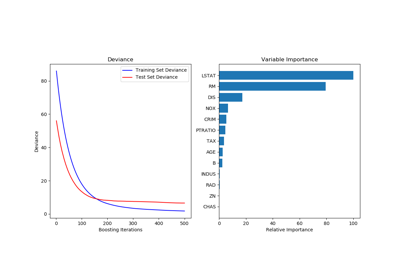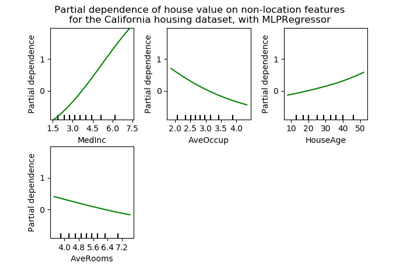# 3.2.4.3.6. sklearn.ensemble.GradientBoostingRegressor¶

class sklearn.ensemble.GradientBoostingRegressor(loss=’ls’, learning_rate=0.1, n_estimators=100, subsample=1.0, criterion=’friedman_mse’, min_samples_split=2, min_samples_leaf=1, min_weight_fraction_leaf=0.0, max_depth=3, min_impurity_decrease=0.0, min_impurity_split=None, init=None, random_state=None, max_features=None, alpha=0.9, verbose=0, max_leaf_nodes=None, warm_start=False, presort=’auto’, validation_fraction=0.1, n_iter_no_change=None, tol=0.0001)[source]

GB builds an additive model in a forward stage-wise fashion; it allows for the optimization of arbitrary differentiable loss functions. In each stage a regression tree is fit on the negative gradient of the given loss function.

Read more in the User Guide.

Parameters: loss : {‘ls’, ‘lad’, ‘huber’, ‘quantile’}, optional (default=’ls’) loss function to be optimized. ‘ls’ refers to least squares regression. ‘lad’ (least absolute deviation) is a highly robust loss function solely based on order information of the input variables. ‘huber’ is a combination of the two. ‘quantile’ allows quantile regression (use alpha to specify the quantile). learning_rate : float, optional (default=0.1) learning rate shrinks the contribution of each tree by learning_rate. There is a trade-off between learning_rate and n_estimators. n_estimators : int (default=100) The number of boosting stages to perform. Gradient boosting is fairly robust to over-fitting so a large number usually results in better performance. subsample : float, optional (default=1.0) The fraction of samples to be used for fitting the individual base learners. If smaller than 1.0 this results in Stochastic Gradient Boosting. subsample interacts with the parameter n_estimators. Choosing subsample < 1.0 leads to a reduction of variance and an increase in bias. criterion : string, optional (default=”friedman_mse”) The function to measure the quality of a split. Supported criteria are “friedman_mse” for the mean squared error with improvement score by Friedman, “mse” for mean squared error, and “mae” for the mean absolute error. The default value of “friedman_mse” is generally the best as it can provide a better approximation in some cases. New in version 0.18. min_samples_split : int, float, optional (default=2) The minimum number of samples required to split an internal node: If int, then consider min_samples_split as the minimum number. If float, then min_samples_split is a fraction and ceil(min_samples_split * n_samples) are the minimum number of samples for each split. Changed in version 0.18: Added float values for fractions. min_samples_leaf : int, float, optional (default=1) The minimum number of samples required to be at a leaf node. A split point at any depth will only be considered if it leaves at least min_samples_leaf training samples in each of the left and right branches. This may have the effect of smoothing the model, especially in regression. If int, then consider min_samples_leaf as the minimum number. If float, then min_samples_leaf is a fraction and ceil(min_samples_leaf * n_samples) are the minimum number of samples for each node. Changed in version 0.18: Added float values for fractions. min_weight_fraction_leaf : float, optional (default=0.) The minimum weighted fraction of the sum total of weights (of all the input samples) required to be at a leaf node. Samples have equal weight when sample_weight is not provided. max_depth : integer, optional (default=3) maximum depth of the individual regression estimators. The maximum depth limits the number of nodes in the tree. Tune this parameter for best performance; the best value depends on the interaction of the input variables. min_impurity_decrease : float, optional (default=0.) A node will be split if this split induces a decrease of the impurity greater than or equal to this value. The weighted impurity decrease equation is the following: N_t / N * (impurity - N_t_R / N_t * right_impurity - N_t_L / N_t * left_impurity)  where N is the total number of samples, N_t is the number of samples at the current node, N_t_L is the number of samples in the left child, and N_t_R is the number of samples in the right child. N, N_t, N_t_R and N_t_L all refer to the weighted sum, if sample_weight is passed. New in version 0.19. min_impurity_split : float, (default=1e-7) Threshold for early stopping in tree growth. A node will split if its impurity is above the threshold, otherwise it is a leaf. Deprecated since version 0.19: min_impurity_split has been deprecated in favor of min_impurity_decrease in 0.19. The default value of min_impurity_split will change from 1e-7 to 0 in 0.23 and it will be removed in 0.25. Use min_impurity_decrease instead. init : estimator or ‘zero’, optional (default=None) An estimator object that is used to compute the initial predictions. init has to provide fit and predict. If ‘zero’, the initial raw predictions are set to zero. By default a DummyEstimator is used, predicting either the average target value (for loss=’ls’), or a quantile for the other losses. random_state : int, RandomState instance or None, optional (default=None) If int, random_state is the seed used by the random number generator; If RandomState instance, random_state is the random number generator; If None, the random number generator is the RandomState instance used by np.random. max_features : int, float, string or None, optional (default=None) The number of features to consider when looking for the best split: If int, then consider max_features features at each split. If float, then max_features is a fraction and int(max_features * n_features) features are considered at each split. If “auto”, then max_features=n_features. If “sqrt”, then max_features=sqrt(n_features). If “log2”, then max_features=log2(n_features). If None, then max_features=n_features. Choosing max_features < n_features leads to a reduction of variance and an increase in bias. Note: the search for a split does not stop until at least one valid partition of the node samples is found, even if it requires to effectively inspect more than max_features features. alpha : float (default=0.9) The alpha-quantile of the huber loss function and the quantile loss function. Only if loss='huber' or loss='quantile'. verbose : int, default: 0 Enable verbose output. If 1 then it prints progress and performance once in a while (the more trees the lower the frequency). If greater than 1 then it prints progress and performance for every tree. max_leaf_nodes : int or None, optional (default=None) Grow trees with max_leaf_nodes in best-first fashion. Best nodes are defined as relative reduction in impurity. If None then unlimited number of leaf nodes. warm_start : bool, default: False When set to True, reuse the solution of the previous call to fit and add more estimators to the ensemble, otherwise, just erase the previous solution. See the Glossary. presort : bool or ‘auto’, optional (default=’auto’) Whether to presort the data to speed up the finding of best splits in fitting. Auto mode by default will use presorting on dense data and default to normal sorting on sparse data. Setting presort to true on sparse data will raise an error. New in version 0.17: optional parameter presort. validation_fraction : float, optional, default 0.1 The proportion of training data to set aside as validation set for early stopping. Must be between 0 and 1. Only used if n_iter_no_change is set to an integer. New in version 0.20. n_iter_no_change : int, default None n_iter_no_change is used to decide if early stopping will be used to terminate training when validation score is not improving. By default it is set to None to disable early stopping. If set to a number, it will set aside validation_fraction size of the training data as validation and terminate training when validation score is not improving in all of the previous n_iter_no_change numbers of iterations. New in version 0.20. tol : float, optional, default 1e-4 Tolerance for the early stopping. When the loss is not improving by at least tol for n_iter_no_change iterations (if set to a number), the training stops. New in version 0.20. feature_importances_ : array, shape (n_features,) Return the feature importances (the higher, the more important the feature). oob_improvement_ : array, shape (n_estimators,) The improvement in loss (= deviance) on the out-of-bag samples relative to the previous iteration. oob_improvement_ is the improvement in loss of the first stage over the init estimator. train_score_ : array, shape (n_estimators,) The i-th score train_score_[i] is the deviance (= loss) of the model at iteration i on the in-bag sample. If subsample == 1 this is the deviance on the training data. loss_ : LossFunction The concrete LossFunction object. init_ : estimator The estimator that provides the initial predictions. Set via the init argument or loss.init_estimator. estimators_ : array of DecisionTreeRegressor, shape (n_estimators, 1) The collection of fitted sub-estimators.

Notes

The features are always randomly permuted at each split. Therefore, the best found split may vary, even with the same training data and max_features=n_features, if the improvement of the criterion is identical for several splits enumerated during the search of the best split. To obtain a deterministic behaviour during fitting, random_state has to be fixed.

References

J. Friedman, Greedy Function Approximation: A Gradient Boosting Machine, The Annals of Statistics, Vol. 29, No. 5, 2001.

1. Friedman, Stochastic Gradient Boosting, 1999

T. Hastie, R. Tibshirani and J. Friedman. Elements of Statistical Learning Ed. 2, Springer, 2009.

Methods

 apply(self, X) Apply trees in the ensemble to X, return leaf indices. fit(self, X, y[, sample_weight, monitor]) Fit the gradient boosting model. get_params(self[, deep]) Get parameters for this estimator. predict(self, X) Predict regression target for X. score(self, X, y[, sample_weight]) Returns the coefficient of determination R^2 of the prediction. set_params(self, \*\*params) Set the parameters of this estimator. staged_predict(self, X) Predict regression target at each stage for X.
__init__(self, loss=’ls’, learning_rate=0.1, n_estimators=100, subsample=1.0, criterion=’friedman_mse’, min_samples_split=2, min_samples_leaf=1, min_weight_fraction_leaf=0.0, max_depth=3, min_impurity_decrease=0.0, min_impurity_split=None, init=None, random_state=None, max_features=None, alpha=0.9, verbose=0, max_leaf_nodes=None, warm_start=False, presort=’auto’, validation_fraction=0.1, n_iter_no_change=None, tol=0.0001)[source]
apply(self, X)[source]

Apply trees in the ensemble to X, return leaf indices.

New in version 0.17.

Parameters: X : {array-like, sparse matrix}, shape (n_samples, n_features) The input samples. Internally, its dtype will be converted to dtype=np.float32. If a sparse matrix is provided, it will be converted to a sparse csr_matrix. X_leaves : array-like, shape (n_samples, n_estimators) For each datapoint x in X and for each tree in the ensemble, return the index of the leaf x ends up in each estimator.
feature_importances_
Return the feature importances (the higher, the more important the
feature).
Returns: feature_importances_ : array, shape (n_features,) The values of this array sum to 1, unless all trees are single node trees consisting of only the root node, in which case it will be an array of zeros.
fit(self, X, y, sample_weight=None, monitor=None)[source]

Parameters: X : {array-like, sparse matrix}, shape (n_samples, n_features) The input samples. Internally, it will be converted to dtype=np.float32 and if a sparse matrix is provided to a sparse csr_matrix. y : array-like, shape (n_samples,) Target values (strings or integers in classification, real numbers in regression) For classification, labels must correspond to classes. sample_weight : array-like, shape (n_samples,) or None Sample weights. If None, then samples are equally weighted. Splits that would create child nodes with net zero or negative weight are ignored while searching for a split in each node. In the case of classification, splits are also ignored if they would result in any single class carrying a negative weight in either child node. monitor : callable, optional The monitor is called after each iteration with the current iteration, a reference to the estimator and the local variables of _fit_stages as keyword arguments callable(i, self, locals()). If the callable returns True the fitting procedure is stopped. The monitor can be used for various things such as computing held-out estimates, early stopping, model introspect, and snapshoting. self : object
get_params(self, deep=True)[source]

Get parameters for this estimator.

Parameters: deep : boolean, optional If True, will return the parameters for this estimator and contained subobjects that are estimators. params : mapping of string to any Parameter names mapped to their values.
predict(self, X)[source]

Predict regression target for X.

Parameters: X : {array-like, sparse matrix}, shape (n_samples, n_features) The input samples. Internally, it will be converted to dtype=np.float32 and if a sparse matrix is provided to a sparse csr_matrix. y : array, shape (n_samples,) The predicted values.
score(self, X, y, sample_weight=None)[source]

Returns the coefficient of determination R^2 of the prediction.

The coefficient R^2 is defined as (1 - u/v), where u is the residual sum of squares ((y_true - y_pred) ** 2).sum() and v is the total sum of squares ((y_true - y_true.mean()) ** 2).sum(). The best possible score is 1.0 and it can be negative (because the model can be arbitrarily worse). A constant model that always predicts the expected value of y, disregarding the input features, would get a R^2 score of 0.0.

Parameters: X : array-like, shape = (n_samples, n_features) Test samples. For some estimators this may be a precomputed kernel matrix instead, shape = (n_samples, n_samples_fitted], where n_samples_fitted is the number of samples used in the fitting for the estimator. y : array-like, shape = (n_samples) or (n_samples, n_outputs) True values for X. sample_weight : array-like, shape = [n_samples], optional Sample weights. score : float R^2 of self.predict(X) wrt. y.

Notes

The R2 score used when calling score on a regressor will use multioutput='uniform_average' from version 0.23 to keep consistent with metrics.r2_score. This will influence the score method of all the multioutput regressors (except for multioutput.MultiOutputRegressor). To specify the default value manually and avoid the warning, please either call metrics.r2_score directly or make a custom scorer with metrics.make_scorer (the built-in scorer 'r2' uses multioutput='uniform_average').

set_params(self, **params)[source]

Set the parameters of this estimator.

The method works on simple estimators as well as on nested objects (such as pipelines). The latter have parameters of the form <component>__<parameter> so that it’s possible to update each component of a nested object.

Returns: self
staged_predict(self, X)[source]

Predict regression target at each stage for X.

This method allows monitoring (i.e. determine error on testing set) after each stage.

Parameters: X : {array-like, sparse matrix}, shape (n_samples, n_features) The input samples. Internally, it will be converted to dtype=np.float32 and if a sparse matrix is provided to a sparse csr_matrix. y : generator of array of shape (n_samples,) The predicted value of the input samples.

## 3.2.4.3.6.1. Examples using sklearn.ensemble.GradientBoostingRegressor¶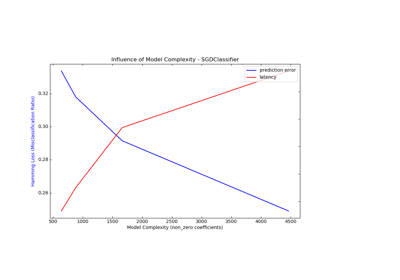Model Complexity Influence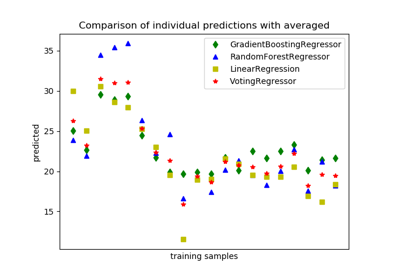Plot individual and voting regression predictions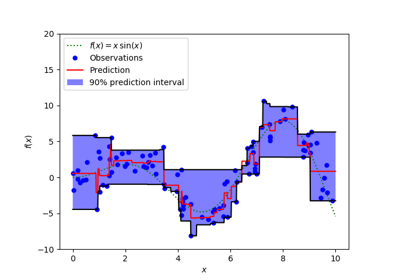Prediction Intervals for Gradient Boosting Regression